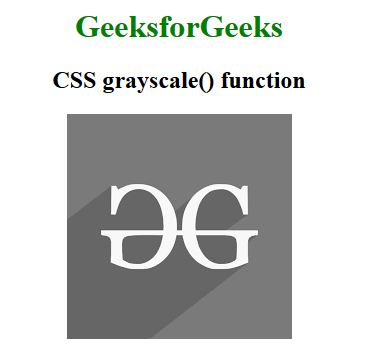# CSS grayscale() Function

The grayscale() function in CSS is an inbuilt function that is used to apply a filter to the image to set the grayscale of the image.

Syntax:

`grayscale( amount )`

Parameters: This function accepts a single parameter amount that holds the value of grayscale. The value of grayscale is set in terms of number and percentage. The value 0% represents the original image while the value between 0% to 100% represents the linear multipliers on the effect.

Example: The below example illustrates the grayscale() function in CSS.

## HTML

 `` `<``html``>`   `<``head``>` `    ``<``title``>CSS grayscale() Function` `    ``<``style``>` `    ``h1 {` `        ``color: green;` `    ``}` `    `  `    ``body {` `        ``text-align: center;` `    ``}` `    `  `    ``.grayscale_effect {` `        ``filter: grayscale(100%);` `    ``}` `    ``` ``   `<``body``>` `    ``<``h1``>GeeksforGeeks` `    ``<``h2``>CSS grayscale() function ` `        ``<``img` `class``=``"grayscale_effect"` `             ``src``=` `"https://media.geeksforgeeks.org/wp-content/cdn-uploads/20190710102234/download3.png"` `             ``alt``=``"GeeksforGeeks logo"``> ` ``   ``

Output:Supported Browsers: The browsers supported by the grayscale() function are listed below: